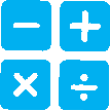###In both science and engineering, mathematics and computation are fundamental tools for representing physical variables and their relationships. They are used for a range of tasks such as constructing simulations; statistically analyzing data; and recognizing, expressing, and applying quantitative relationships.

Primary (K-2)

Mathematical and computational thinking at the K–2 level builds on prior experience and progresses to recognizing that mathematics can be used to describe the natural and designed world.

• Decide when to use qualitative vs. quantitative data.
• Use counting and numbers to identify and describe patterns in the natural and designed world(s).
• Describe, measure, and/or compare quantitative attributes of different objects and display the data using simple graphs.
• Use quantitative data to compare two alternative solutions to a problem.

Elementary (3-5)

Mathematical and computational thinking at the 3–5 level builds on K–2 experiences and progresses to extending quantitative measurements to a variety of physical properties and using computation and mathematics to analyze data and compare alternative design solutions.

• Decide if qualitative or quantitative data are best to determine whether a proposed object or tool meets criteria for success.
• Organize simple data sets to reveal patterns that suggest relationships.
• Describe, measure, estimate, and/or graph quantities such as area, volume, weight, and time to address scientific and engineering questions and problems.
• Create and/or use graphs and/or charts generated from simple algorithms to compare alternative solutions to an engineering problem.

Middle (6-8)

Mathematical and computational thinking at the 6–8 level builds on K–5 experiences and progresses to identifying patterns in large data sets and using mathematical concepts to support explanations and arguments.

• Use digital tools (e.g., computers) to analyze very large data sets for patterns and trends.
• Use mathematical representations to describe and/or support scientific conclusions and design solutions.
• Create algorithms (a series of ordered steps) to solve a problem.
• Apply mathematical concepts and/or processes (such as ratio, rate, percent, basic operations, and simple algebra) to scientific and engineering questions and problems.
• Use digital tools and/or mathematical concepts and arguments to test and compare proposed solutions to an engineering design problem.

High (9-12)

Mathematical and computational thinking in 9–12 builds on K–8 experiences and progresses to using algebraic thinking and analysis, a range of linear and nonlinear functions including trigonometric functions, exponential and logarithms, and computational tools for statistical analysis to analyze, represent, and model data. Simple computational simulations are created and used based on mathematical models of basic assumption.

• Create and/or revise a computational model or simulation of a phenomenon, designed device, process, or system.
• Use mathematical, computational, and/or algorithmic representations of phenomena or design solutions to describe and/or support claims and/or explanations.
• Apply techniques of algebra and functions to represent and solve scientific and engineering problems.
• Use simple limit cases to test mathematical expressions, computer programs, algorithms, or simulations of a process or system to see if a model “makes sense” by comparing the outcomes with what is known about the real world.
• Apply ratios, rates, percentages, and unit conversions in the context of complicated measurement problems involving quantities with derived or compound units (such as mg/mL, kg/m3, acre-feet, etc.)

Although there are differences in how mathematics and computational thinking are applied in science and in engineering, mathematics often brings these two fields together by enabling engineers to apply the mathematical form of scientific theories and by enabling scientists to use powerful information technologies designed by engineers. Both kinds of professionals can thereby accomplish investigations and analyses and build complex models, which might otherwise be out of the question.

Students are expected to use mathematics to represent physical variables and their relationships, and to make quantitative predictions. Other applications of mathematics in science and engineering include logic, geometry, and at the highest levels, calculus. Computers and digital tools can enhance the power of mathematics by automating calculations, approximating solutions to problems that cannot be calculated precisely, and analyzing large data sets available to identify meaningful patterns. Students are expected to use laboratory tools connected to computers for observing, measuring, recording, and processing data. Students are also expected to engage in computational thinking, which involves strategies for organizing and searching data, creating sequences of steps called algorithms, and using and developing new simulations of natural and designed systems. Mathematics is a tool that is key to understanding science. As such, classroom instruction must include critical skills of mathematics. The ND Science Standards displays many of those skills through the performance expectations, but classroom instruction should enhance all of science through the use of quality mathematical and computational thinking.